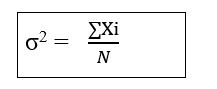### Sample Variance

• date 25th June, 2019 |
• by Prwatech |

# Sample Variance Tutorial

Sample Variance tutorial: Here, one can learn how to calculate the sample variance in data science with example. Before we proceed, we recommend you to go through the previous blog in this series on how to calculate mean, median and mode.

Are you the one who is looking to learn about sample variance in data science using python? Or Are you the one who is looking for the best platform to learn Data science tutorial?  Or Are you the one who is dreaming to become an expert data scientist? Then stop dreaming yourself, start taking Data Science training from Prwatech, who can help you to guide and offer excellent training with highly skilled expert trainers with the 100% placement.

## What is the Sample Variance?

The sample variance, s2, is used to calculate how varied a sample is. A sample is a select number of items taken from a population. For example, if you are measuring Indian people’s weights, it wouldn’t be feasible for you to measure the weights of every person in the population. The solution is to take a sample of the population, say 10000 people, and use that sample size to estimate the actual weights of the whole population. The variance helps you to figure out how to spread out your weights are.

## Why sample variance used for data science?

While the variance is useful in a mathematical sense, it won’t actually give you any information that you can use. For example, if you take a sample population of weights, you might end up with a variance of 9801. That might leave you scratching your head about why you’re calculating it in the first place! The answer is, you can use the variance to figure out the standard deviation — a much better measure of how to spread out your weights are. In order to get the standard deviation, take the square root of the sample variance:

√9801 = 99.

The standard deviation, in combination with the mean, will tell you what the majority of people weigh. For example, if your mean is 150 pounds and your standard deviation is 99 pounds, the majority of people weigh between 51 pounds (mean-99) and 249 pounds (mean+99).

### How to Calculate Sample Variance in data science?

Step1) Square all the Items in the Data Set

Step2)  Take summation of the above the Squares

Step3)   Divide the above result by Number of Items (N).

## Sample Variance Formula## Sample Variance Example

 3 5 6 4 8 10 6 7 2 1

Now Make Squares of all the terms:

 9 25 36 16 64 100 36 49 4 1

Now add all the above terms:

∑Xi= 9+25+36+16+64+100+36+49+4+1

∑Xi=340

σ² =340/10

σ² =34

In this Sample variance tutorial, we have covered concepts of what is sample variance, its use in data science and how to calculate sample variance with examples. This will get you a clear idea about Sample Variance in Data Science.

Ready to take the first step towards a career in Data Science? Or if you are considering a career in Data Science. Then get an advanced Data science course from Data Science Training institute in Bangalore by skilled trainers like a Pro.

We hope you liked our article on the Sample Variance tutorial. Share your feedback with your comments.

### Quick Support